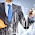# Structures Simplified

## "Let's simplify the art of Structural Engineering"

August 06, 2020 Posted by Parishith Jayan

## What happens to the load applied to a structure?

Whatever the load which acts on the structure ultimately tries to reach the ground from its point of application. Is not it?

This path is called “Load Path”.

Just imagine, you are departing from your workplace and commuting to your home.

What will be the first thing you do; you find your mode of transportation (say bike) and tries to figure out the route to reach your destination, right? There may be several routes for you to consider.

Similarly, for a load to reach the ground there may be more than one load path to consider.

After which, what you do, will choose the quickest way to travel. Correct?

The loads which are applied to the structure behave exactly the same. It always tries to find the shortest path to reach the ground.

Let’s see this with an example. The beam which is shown in the figure below is a simply supported beam of 3m length resting over a column. A load of 10kN acts at 1m from its left support.

What is the possible load path for this simple structure?

The load can move towards its left and reach the beam-column joint through which it gets transferred to the column, then from the column, it can reach the ground.

A similar path on the right is also possible.

But which is the shortest path? Transferring through the left side right. Yes, that is what happens. A major amount of load gets transferred to the ground through left support. This can be realized by calculating the reaction at supports S1 and S2.

Applying equilibrium equations and determining support reaction yields the value of 6.66kN for support S1 and 3.33kN for support S2 respectively.

What is so important in this? Yeah, the load is taking the shortest path. Why we need to know about which way the load is moving?

1. It is important because each element along the load path must have sufficient strength and stiffness to safely transfer the load. This is where design gets in. So, without understanding the load path, an engineer could not design a structure.

2. Secondly, a load gets transfer from one member to another through a connection. The load path plays a major role in determining what are the forces to consider while designing the connection.

Let’s look into the following figure to understand the importance of a load path in designing.

It is a gusset plate connection, connecting the floor brace to the column.

How the load is getting transferred here? Or more precisely what is the load-path?

If we draw a simple line diagram of the above connection, it will look like a crosshair with one diagonal, and this leads to the misconception that the load-path will be directly from the bracing member to the column through connection.

But the actual load path is different from what we assume.

The load from bracing moves to the beam through a gusset plate connection, and from the beam it is transferred to the column.

If we didn’t consider this load while designing the beam, then it will lead to the failure of the section due to this unexpected load. This simple example portrays the importance of understanding the load-path to reach a safe design.

Understanding how load travels through a structure is the key to optimal design.

If you guys want to know more regarding the behavior of a structure. Let me know in the comment.

#### 1 comment:

1.yes sir, its intersting